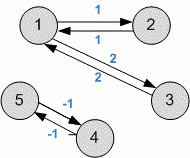Problems

# Floyd - existence

Time limit 1 second
Memory limit 128 MiB

Given a directed weighted graph. Using its weighted matrix, determine for each pair of vertices does there exist the shortest path between them or not.

The shortest path may not exist for two reasons:

• There is no way.

• There is a way of arbitrarily small weight.

## Input data

The first line contains the number of vertices n (1n100). The next n lines contains n numbers that describe the adjacency matrix (j-th of the i-th row corresponds to the weight of the edge from vertex i to vertex j). The number 0 in this matrix represents no edge, and any other number - the existence of an edge of corresponding weight. All the numbers do not exceed 100 by absolute value.

## Output data

Print n rows with n numbers: the j-th number in the i-th row must be 0 if the path from i to j does not exist, 1 if there is a shortest path, and 2 if there is a path of arbitrarily small weight.## Examples

Input example #1
5
0 1 2 0 0
1 0 3 0 0
2 3 0 0 0
0 0 0 0 -1
0 0 0 -1 0

Output example #1
1 1 1 0 0
1 1 1 0 0
1 1 1 0 0
0 0 0 2 2
0 0 0 2 2